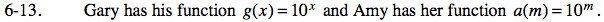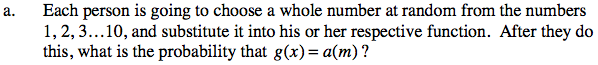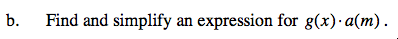### Home > CAAC > Chapter 13 > Lesson 13.IF1-S > Problem6-13

6-13.Notice that g(x) and a(m) are equivalent functions, what is the probability that Gary and Amy pick the same number?

Assume Gary has chosen a number. How many chances does Amy have to pick the same number?
Out of how many choices?Remember the exponent rules.

10x + m